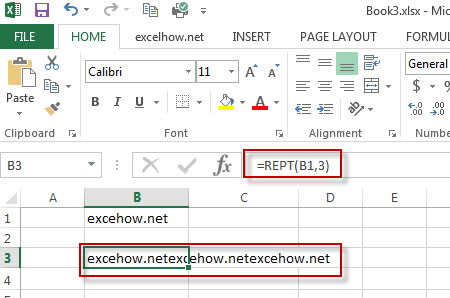# Excel Rept Function

This post will guide you how to use Excel REPT function with syntax and examples in Microsoft excel.

### Description

The Excel REPT function repeats a text string a specified number of times.

The REPT function is a build-in function in Microsoft Excel and it is categorized as a Text Function.

The REPT function is available in Excel 2016, Excel 2013, Excel 2010, Excel 2007, Excel 2003, Excel XP, Excel 2000, Excel 2011 for Mac.

### Syntax

The syntax of the REPT function is as below:

= REPT  (text, number_times)

Where the REPT function arguments are:
Text -This is a required argument. The text string that you want to repeat.
Number_times – This is an required argument.  The number of times that you want to repeat
Note: If number_times is zero, the REPT function will return empty text. And If number_times value is not an integer, it is truncated to an integer value.

### Example

The below examples will show you how to use Excel REPT Text function to repeat a text string a given number of times.

#1 To repeat a text string in B1 cell 3 times, just using the following formula:= REPT (B1,3).